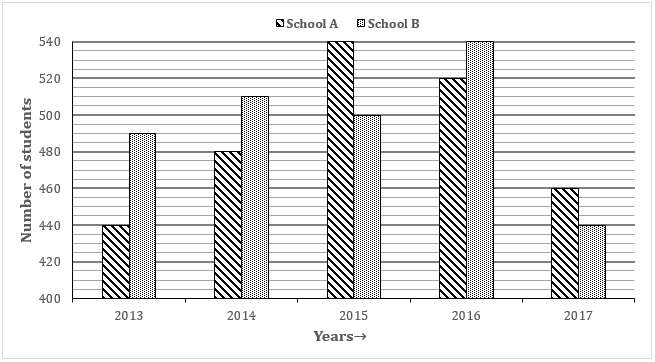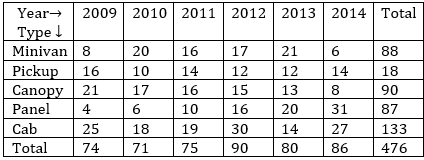# IBPS RRB 2019 Prelims Quantitative Aptitude: PO/Clerk | 25th JulyIBPS RRB Quantitative Aptitude Quiz

Here, we are providing IBPS RRB Study Plan as there is left not enough time to deal in details. The questions asked in the quantitative aptitude section are calculative and very time-consuming. But once dealt with proper strategy, speed, and accuracy, this section can get you the maximum marks in the examination. Following is the Quantitative Aptitude Quiz to help you practice with the best of latest pattern questions.

Directions (1-5): - Bar graph given below shows number of students passed in an exam from school A and B in five different years. Study the bar graph carefully and answer the following questions.Q1. In 2016, ratio between boys passed to girls passed from school ‘A’ and School ‘B’ are 8 : 5 and 16 : 11 respectively. Find the ratio between boys passed from school ‘A’ and ‘B’ together in 2016 to girls passed from school ‘A’ and ‘B’ together in 2016.

23 : 31
32 : 21
29 : 17
25 : 37
3 : 4

Q2. If in 2014, 96% and 85% students passed from school A and B respectively out of total students appeared, then find the average number of failed students from school A and B together in 2014.

20
60
75
55
65

Q3. Students passed from school ‘A’ in 2013, 2015, 2017 together is how much less than students passed from school B in 2014, 2016, 2017 together.

50
40
60
70
80
Solution:

Students passed from school A in 2013, 2015, 2017 = 440 + 540 + 460 = 1440

Students passed from school B in 2014, 2016 and 2017 = 510 + 540 + 440 = 1490

Required difference = 1490 – 1440 = 50

Q4. In 2014, total 600 students appeared from school ‘A’ for exam in which ratio between boys and girls is 7 : 5. If out of total girls appeared in exam 90% of girls passed, then find the number of boys passed from school ‘A’ is what percent of the total number of boys appeared in the exam?

169/2%
763/8%
510/7%
76%
528/7%

Q5. Find the ratio between total number of students passed from school ‘A’ in 2014 and 2015 together to total number of students appeared from school B in 2016 and 2017 together.

51 : 49
49 : 51
47 : 45
53 : 48
Cannot be determined
Solution:

Total number of students from school B cannot be determined as data is not given.

Directions (6-10): The table below shows production of five types of Trucks by a company in the years 2009 to 2014. Study the table and answer questions.

Production of trucks by a companyQ6. In which year the production of trucks of all types taken together was approximately equal to the average of the total production during the period?

2009
2011
2013
2014
None of these
Solution:

Average of the total production during the period = 476/5 ≈ 95 Which is not equal to the total production in any of the given years.

Q7. In which year, the total production of trucks of types of Minivan and Pickup together was equal to the total production of trucks of types Canopy and Panel together.

2010
2011
2014
2013
2012
Solution:

Q8. During the period 2009-14, in which type of trucks was a continuous increase in production?

Minivan
Pickup
Canopy
Panel
Cab
Solution:

Q9. The production of which type of trucks was 25% of the total production of all types of trucks during 2013?

Panel
Canopy
Pickup
Minivan
Cab
Solution:

25% of 80 = 20 = production of Panel in 2013.

Q10. The per cent increase in total production of all types of trucks in 2012 to that in 2011 was:

15
20
25
30
35

Directions (11-15): Dominos prepares Pizzas of three different types – Cheese, Onion and Chicken. The production of the three types over a period of six months has been expressed in the bar-graph provided below. Study the graph and answer the questions based on it.

Q11. For which of the following months the percentage of rise/fall in order from the previous month is the maximum for the Onion flavor?

February
March
April
May
June
Solution:

Q12. For which type of pizza was the average order maximum in the given period?

Cheese only
Onion only
Chicken only
Cheese and Onion
Cheese and Chicken

Q13. The total order of Chicken type in March and April together is what percentage of the total order of Cheese type in January and February together?

96.67%
102.25%
115.57%
120%
133.33%

Q14. What is the difference between the average order of Cheese type in January, February and March and the average order of Onion type in April, May and June?

50,000 orders
80,000 orders
2,40,000 orders
3,30,000 orders
5,00,000 orders

Q15. What was the approximate decline in the Order of Chicken type in June as compared to the Order in April?

50%
42%
33%
25%
22.5%
Solution:

You May also like to Read: